# Context

Retail dataset of a global superstore for 4 years.
Perform EDA and Predict the sales of the next 6 days from the last date of the Training dataset!

# Content

Time series analysis deals with time series based data to extract patterns for predictions and other characteristics of the data. It uses a model for forecasting future values in a small time frame based on previous observations. It is widely used for non-stationary data, such as economic data, weather data, stock prices, and retail sales forecasting.

Seasonal Autoregressive Integrated Moving Average, SARIMA or Seasonal ARIMA, is an extension of ARIMA that explicitly supports univariate time series data with a seasonal component.

It adds three new hyperparameters to specify the autoregression (AR), differencing (I) and moving average (MA) for the seasonal component of the series, as well as an additional parameter for the period of the seasonality.

# How to Configure SARIMA

Configuring a SARIMA requires selecting hyperparameters for both the trend and seasonal elements of the series.

# Trend Elements

There are three trend elements that require configuration.

They are the same as the ARIMA model; specifically:

• p: Trend autoregression order.
• d: Trend difference order.
• q: Trend moving average order.

# Seasonal Elements

There are four seasonal elements that are not part of ARIMA that must be configured; they are:

• P: Seasonal autoregressive order.
• D: Seasonal difference order.
• Q: Seasonal moving average order.
• m: The number of time steps for a single seasonal period.

Together, the notation for an SARIMA model is specified as:

SARIMA(p,d,q)(P,D,Q)m

# Dataset

The dataset is easy to understand and is self-explanatory.

# Importing the Libraries

`import numpy as npimport pandas as pdimport matplotlib.pyplot as plt%matplotlib inlinefrom statsmodels.tsa.statespace.sarimax import SARIMAXimport warningswarnings.filterwarnings('ignore')`

`data = pd.read_csv("../input/superstore-data-demand-forecasting/superstore.csv")data = data.drop_duplicates()data.shape(9800, 22)data.columnsIndex(['Order ID', 'Order Date', 'Ship Date', 'Ship Mode', 'Customer ID',       'Customer Name', 'Segment', 'Country', 'City', 'State', 'Postal Code',       'Region', 'Product ID', 'Category', 'Sub-Category', 'Product Name',       'Sales', 'Quantity', 'Discount', 'Profit', 'Price'],      dtype='object')`

# Exploratory Data Analysis

`def plotbarcharts(dataset,columns):    %matplotlib inline    fig,subplot = plt.subplots(nrows=1,ncols=len(columns),figsize=(18,5))    fig.suptitle('Bar Chart for' + str(columns))    for columnname,plotnumber in zip(columns,range(len(columns))):        dataset.groupby(columnname).size().plot(kind='bar',ax=subplot[plotnumber])columnsList1 = ['Ship Mode','Region']columnsList2 = ['Region','Category','Sub-Category']plotbarcharts(data,columnsList1)`
• Most of the orders are from Standard Class of Ship Mode.
• Most of the orders are coming from west region followed by east region.
`plotbarcharts(data,columnsList2)`
• Office Supplies has the highest count among categories.
• Binders have the highest count followed by Papers and Furnishing in sub-category.
`data.groupby(['State']).size().plot(kind='bar',figsize=(18,8))`
• Most of the orders are coming from California followed by New York and Texas.
`data.groupby(['Month']).size().plot(kind='bar')`
• Most of the orders are from November month.
`data.groupby(['Year']).size().plot(kind='bar')`
• Year 2018 received the highest number of orders.
`data.set_index("Order Date", inplace = True)data['Sales'].plot()`

# Aggregating the sales quantity for each month for all categories

`pd.crosstab(columns=data['Month'],            index=data['Year'],            values=data['Sales'],            aggfunc='sum')import matplotlib.pyplot as pltSalesQuantitiy=pd.crosstab(columns=data['Year'],            index=data['Month'],            values=data['Sales'],            aggfunc='sum').melt()['value']MonthNames=['Jan','Feb','Mar','Apr','May', 'Jun', 'Jul', 'Aug', 'Sep','Oct','Nov','Dec']*4# Plotting the sales%matplotlib inlineSalesQuantitiy.plot(kind='line', figsize=(16,5), title='Total Sales Quantity per month')# Setting the x-axis labelsplotLabels=plt.xticks(np.arange(0,48,1),MonthNames, rotation=30)`
• There is a clear seasonal pattern in the dataset.

# Seasonal Decompose

`from statsmodels.tsa.seasonal import seasonal_decomposeseries = SalesQuantitiy.valuesresult = seasonal_decompose(series, model='additive', freq=12)result.plot()CurrentFig=plt.gcf()CurrentFig.set_size_inches(11,8)plt.show()`

# Applying SARIMAX

`SarimaxModel = model = SARIMAX(SalesQuantitiy,                          order = (5, 1, 10),                          seasonal_order =(1, 0, 0, 12))SalesModel = SarimaxModel.fit()`

# Forecasting for the next 6 months

`forecast = SalesModel.predict(start = 0,                          end = (len(SalesQuantitiy)) + 6,                          typ = 'levels').rename('Forecast')print("Next Six Month Forecast:",forecast[-6:])`

# Plotting the forecasted values

`SalesQuantitiy.plot(figsize = (18, 5), legend = True, title='Time Series Sales Forecasts')forecast.plot(legend = True, figsize=(18,5))`

# Measuring the Accuracy of the model

`MAPE=np.mean(abs(SalesQuantitiy-forecast)/SalesQuantitiy)*100print('#### Accuracy of model:', round(100-MAPE,2), '####')Printing month names in X-AxisMonthNames=MonthNames+MonthNames[0:6]plotLabels=plt.xticks(np.arange(0,54,1),MonthNames, rotation=30)`

# Results

With SARIMAX we are getting an accuracy of 76%, by further applying hyperparameter tuning we can improve the accuracy and also try different time series techniques like Facebook Prophet, Autoregressive Moving Average (ARMA), Autoregressive Integrated Moving Average (ARIMA) etc.

# Hope you liked the analysis!

https://www.kaggle.com/bravehart101/sample-supermarket-dataset/code

Ratul | Notebooks Contributor | Kaggle

--

--

--

Software Engineer at Cyient | Data Science | Analytics | ML | AI | Deep Learning | NLP

Love podcasts or audiobooks? Learn on the go with our new app.

## Step 1: The design journey begins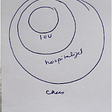## The Difference Between Pre-Event Predictability and Post-Event Explainability## Network and Interconnection in Python Maps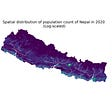## Food Delivery Financial model For Click & Collect Business## Plotting a map of London Crime Data using R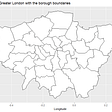## Project Ideas## 3 Awesome Python Libraries That You Should Know About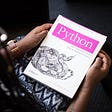## How Russia’s World Cup Ad Took It to a New Level## Ratul Ghosh

Software Engineer at Cyient | Data Science | Analytics | ML | AI | Deep Learning | NLP

## Time Series Analysis on Daily Revenue Data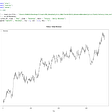## Time Series Forecasting While Considering Holidays with FBProphet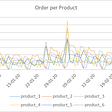## CAN you tell the Future? Humm, Maybe …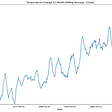## Dealing with Time-series Data issues